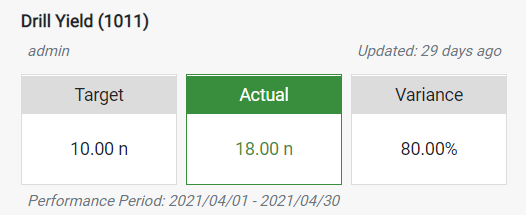• "admin" - This is the owner of the KPI.
• "Updated: 29 days ago" - This period is determined by the latest Actual value date .
• "Performance Period: 2021/04/01 - 2021/04/30"- This is the period used to calculate the Target and Actual values displayed(The performance period of this KPI is set to Last Month).
• Using the determined Actual and Target values the variance is then calculated as follows taking into account the trend of the KPI(e.g. This is an upwards KPI):

The variance will be Actual -Target = 18 - 10 = 8(This is the absolute variance) meaning the variable percentage will be (Actual-Target)/Target = (18-10)/10 = 0.8 = 80%
• The status of the KPI is then determined as follows
• The KPI will be Green if the Variance is positive. (Variance >=0 )
• The KPI will be Amber if the Variance percentage is within the Warning Levels.
• Otherwise Red.